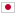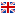G - Additions/### Problem Statement

You are given an integer $N$ and a string consisting of + and digits. You are asked to transform the string into a valid formula whose calculation result is smaller than or equal to $N$ by modifying some characters. Here, you replace one character with another character any number of times, and the converted string should still consist of + and digits. Note that leading zeros and unary positive are prohibited.

For instance, 0123+456 is assumed as invalid because leading zero is prohibited. Similarly, +1+2 and 2++3 are also invalid as they each contain a unary expression. On the other hand, 12345, 0+1+2 and 1234+0+0 are all valid.

Your task is to find the minimum number of the replaced characters. If there is no way to make a valid formula smaller than or equal to $N$, output $-1$ instead of the number of the replaced characters.

### Input

The input consists of a single test case in the following format.

$N$ $S$

The first line contains an integer $N$, which is the upper limit of the formula ($1 \le N \le 10^9$). The second line contains a string $S$, which consists of + and digits and whose length is between $1$ and $1{,}000$, inclusive. Note that it is not guaranteed that initially $S$ is a valid formula.

### Output

Output the minimized number of the replaced characters. If there is no way to replace, output $-1$ instead.

### Sample Input 1

100
+123


### Output for Sample Input 1

2


### Sample Input 2

10
+123


### Output for Sample Input 2

4


### Sample Input 3

1
+123


### Output for Sample Input 3

-1


### Sample Input 4

10
++1+


### Output for Sample Input 4

2


### Sample Input 5

2000
1234++7890


### Output for Sample Input 5

2


In the first example, you can modify the first two characters and make a formula 1+23, for instance. In the second example, you should make 0+10 or 10+0 by replacing all the characters. In the third example, you cannot make any valid formula less than or equal to $1$.